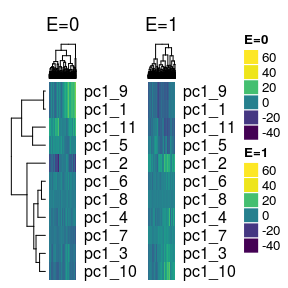# Introduction to eclust

#### 2017-01-25

Notes:

• This vignette was built with R markdown and knitr. The source code for this vignette can be found here.

• This is a brief introduction to the eclust package. This package clusters gene expression or DNA methylation data that is sensitive to environmental exposures. It is the companion package to the paper

Bhatnagar, SR., Yang, Y., Blanchette, M., Bouchard, L., Khundrakpam, B., Evans, A., Greenwood, CMT. (2017+). An analytic approach for interpretable predictive models in high dimensional data, in the presence of interactions with exposures. Preprint

The following figure is an overview of what the ECLUST method does: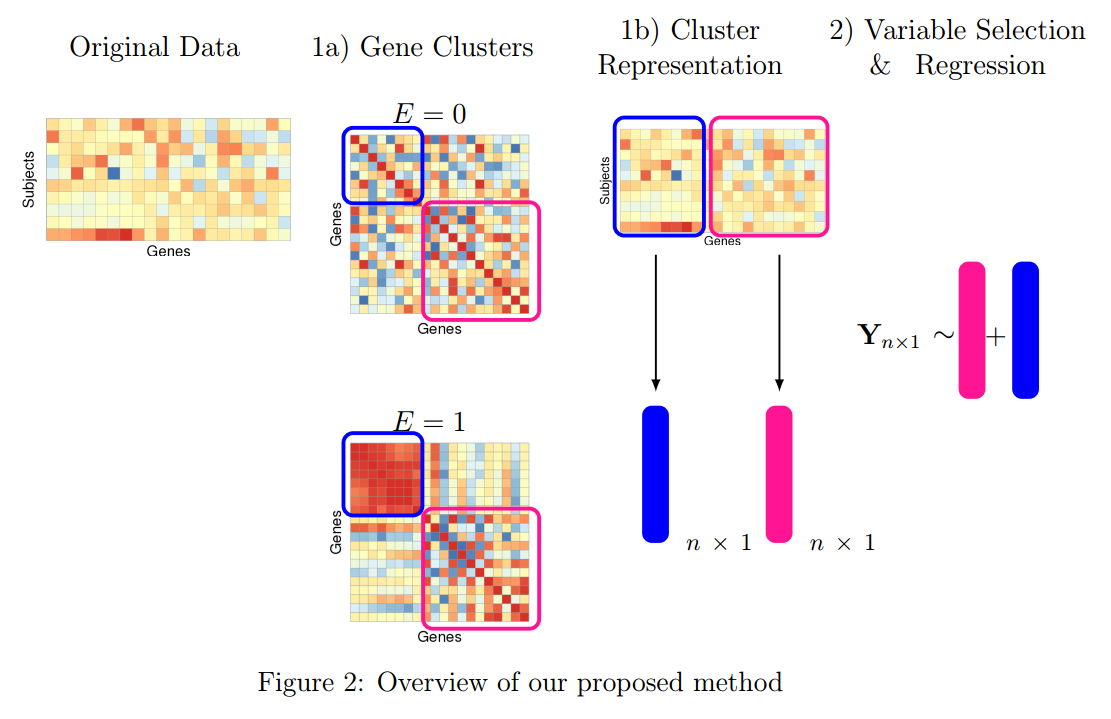# 1 Installation

You can install eclust from CRAN:

install.packages("eclust")

Alternatively, you can install the development version of eclust from GitHub with:

install.packages("pacman")
pacman::p_install_gh("sahirbhatnagar/eclust")

# 2 Data

There are two datasets included in this package that can be loaded into your R session via data(tcgaov) and data(simdata):

1. tcgaov: A dataset containing a subset of the TCGA mRNA Ovarian serous cystadenocarcinoma data generated using Affymetrix HTHGU133a arrays. 511 samples (rows) and 881 genes (columns).
2. simdata: A dataset containing simulated data for example use of the eclust package functions. A matrix with 100 rows 500 genes, a continuous response Y and a binary environment vector E.

# 3 Overview of Functions

This package has three sets of functions starting with either r_, s_ or u_

1. r_ (real data functions): related to analysis of real data. Most users will apply this set of functions to their data.
function.name
r_cluster_data
r_prepare_data
1. s_ (simulation functions): related to the simulations conducted in the paper. There are functions to simulate data, run the analyses on these data, and output performance metrics.
function.name
s_generate_data
s_generate_data_mars
s_mars_clust
s_mars_separate
s_modules
s_pen_clust
s_pen_separate
s_response
s_response_mars
1. u_ (utility functions): functions that are used by both simulation and real data analysis functions. Not really meant to be called by the user.
function.name
u_cluster_similarity
u_extract_selected_earth
u_extract_summary
u_fisherZ

# 4 Real data analysis functions (r_)

We will use the data(tcgaov) dataset included in this package, which contains a subset of the TCGA mRNA Ovarian serous cystadenocarcinoma data generated using Affymetrix HTHGU133a arrays. See ?tcgaov for details about the data.

In the example below we use the r_cluster_data to create the environment based clusters, and their summaries. We then use the r_prepare_data function to get it into proper form for regression routines such as earth::earth, glmnet::cv.glmnet, and ncvreg::ncvreg.

## 4.1 Extract the relevant data

# load the data
data("tcgaov")
tcgaov[1:5,1:6, with = FALSE]
##              rn subtype E status   OS  ABCA8
## 1: TCGA-04-1331       4 1      1 1336 3.6848
## 2: TCGA-04-1332       1 0      1 1247 7.8930
## 3: TCGA-04-1335       3 1      1   55 5.1932
## 4: TCGA-04-1336       3 1      0 1495 3.0554
## 5: TCGA-04-1337       1 0      1   61 3.1494
# use log survival as the response
Y <- log(tcgaov[["OS"]])

# specify the environment variable
E <- tcgaov[["E"]]

# specify the matrix of genes only
genes <- as.matrix(tcgaov[,-c("OS","rn","subtype","E","status"),with = FALSE])

# for this example the training set will be all subjects.
# change p argument to create a train and test set.
trainIndex <- drop(caret::createDataPartition(Y, p = 1, list = FALSE, times = 1))
testIndex <- trainIndex

## 4.2 Cluster the data and calculate cluster representations

We cluster the genes using the correlation matrix (specified by cluster_distance = "corr") and the difference of the exposure dependent correlation matrices (specified by eclust_distance = "diffcorr")

cluster_res <- r_cluster_data(data = genes,
response = Y,
exposure = E,
train_index = trainIndex,
test_index = testIndex,
cluster_distance = "corr",
eclust_distance = "diffcorr",
measure_distance = "euclidean",
clustMethod = "hclust",
cutMethod = "dynamic",
method = "average",
nPC = 1,
minimum_cluster_size = 30)
## 
##  ..cutHeight not given, setting it to 10.9  ===>  99% of the (truncated) height range in dendro.
##  ..done.
## Calculating number of environment clusters based on diffcorr
##  ..cutHeight not given, setting it to 0.923  ===>  99% of the (truncated) height range in dendro.
##  ..done.
## There are 7 clusters derived from the corr similarity matrix
## There are 4 clusters derived from the diffcorr environment similarity matrix
## There are a total of 11 clusters derived from the corr
##                   similarity matrix and the diffcorr environment similarity matrix
# the number of clusters determined by the similarity matrices specified
# in the cluster_distance and eclust_distance arguments. This will always be larger
# than cluster_res$clustersAll$nclusters which is based on the similarity matrix
# specified in the cluster_distance argument
cluster_res$clustersAddon$nclusters
##  11
# the number of clusters determined by the similarity matrices specified
# in the cluster_distance argument only
cluster_res$clustersAll$nclusters
##  7
# what's in the cluster_res object
names(cluster_res)
##  "clustersAddon"               "clustersAll"
##  "etrain"                      "cluster_distance_similarity"
##  "clustersAllMembership"       "clustersEclustMembership"

## 4.3 Prepare data for input in any regression routine

Now we use the r_prepare_data function, where we are using the average expression from each cluster as feaand their interaction with E as features in the regression model:

# prepare data for use with earth function
avg_eclust_interaction <- r_prepare_data(data = cbind(cluster_res$clustersAddon$averageExpr,
Y = Y[trainIndex],
E = E[trainIndex]),
response = "Y", exposure = "E")

head(avg_eclust_interaction[["X"]])
##     avg1   avg2   avg3   avg4   avg5   avg6   avg7   avg8   avg9  avg10
## 1 5.5673 4.6034 5.6459 5.4635 6.3307 5.0177 5.7237 4.5379 5.5046 5.7694
## 2 6.4160 5.1355 5.9154 5.4130 6.5398 5.5142 6.3306 4.9493 6.1950 6.1192
## 3 4.7517 4.4289 6.0642 4.5535 5.6475 5.4349 4.8541 4.9238 4.8293 5.6760
## 4 4.4881 5.3601 6.1843 4.4409 7.7475 5.4752 5.5094 4.9190 4.8552 6.1171
## 5 6.6613 5.3162 5.8736 4.9512 5.7700 5.2547 6.8684 4.6187 6.2829 5.8145
## 6 6.2069 5.7833 6.0176 4.8622 6.0019 5.3701 6.6806 4.4445 6.0005 6.0444
##    avg11 E avg1:E avg2:E avg3:E avg4:E avg5:E avg6:E avg7:E avg8:E avg9:E
## 1 4.6050 1 5.5673 4.6034 5.6459 5.4635 6.3307 5.0177 5.7237 4.5379 5.5046
## 2 4.7873 0 0.0000 0.0000 0.0000 0.0000 0.0000 0.0000 0.0000 0.0000 0.0000
## 3 4.2279 1 4.7517 4.4289 6.0642 4.5535 5.6475 5.4349 4.8541 4.9238 4.8293
## 4 4.7544 1 4.4881 5.3601 6.1843 4.4409 7.7475 5.4752 5.5094 4.9190 4.8552
## 5 5.0481 0 0.0000 0.0000 0.0000 0.0000 0.0000 0.0000 0.0000 0.0000 0.0000
## 6 5.1903 0 0.0000 0.0000 0.0000 0.0000 0.0000 0.0000 0.0000 0.0000 0.0000
##   avg10:E avg11:E
## 1  5.7694  4.6050
## 2  0.0000  0.0000
## 3  5.6760  4.2279
## 4  6.1171  4.7544
## 5  0.0000  0.0000
## 6  0.0000  0.0000

## 4.4 Fit a regression model

At this stage, you can decide which regression model to use. Here we choose the MARS model from the earth package, but you may choose regression models from any number of packages (e.g. see the extensive list of models of models available in the caret package).

# install and load earth package

fit_earth <- earth::earth(x = avg_eclust_interaction[["X"]], y = avg_eclust_interaction[["Y"]],
pmethod = "backward",
keepxy = TRUE, degree = 2, trace = 1, nk = 1000)
## x[511,23] with colnames avg1 avg2 avg3 avg4 avg5 avg6 avg7 avg8 avg9 avg10 avg11 ...
## y[511,1] with colname avg_eclust_interaction[["Y"]]
## Forward pass term 1, 2, 4, 6, 8, 10, 12, 14, 16, 18, 20, 22, 24, 26, 28,
##      30, 32, 34, 36, 38
## RSq changed by less than 0.001 at 37 terms, 35 terms used (DeltaRSq 0)
## After forward pass GRSq -0.176 RSq 0.193
## Prune method "backward" penalty 3 nprune null: selected 6 of 35 terms, and 4 of 23 preds
## After pruning pass GRSq 0.01 RSq 0.0579
coef(fit_earth)
##                         (Intercept) h(avg9-5.58701) * h(6.54361-avg5:E)
##                              6.7185                              1.0701
##   h(avg1-5.99657) * h(avg7-6.84195)   h(avg1-5.99657) * h(avg7-6.70675)
##                             67.8507                            -39.7866
##   h(avg1-5.99657) * h(avg7-6.98039)                     h(avg9-5.50456)
##                            -28.7202                             -5.2938

You can also install the plotmo package to visualise the relationships between the hinge functions and the response using plotmo::plotmo(fit_earth).

## 4.5 Determine the features that have been selected

The u_extract_selected_earth is a utility function in this package to extract the selected predictors from the MARS model:

u_extract_selected_earth(fit_earth)
##  "avg1"   "avg7"   "avg9"   "avg5:E"

We that genes in clusters 1, 7 and 9 were selected. We also see that the interaction between the genes in cluster 5 and the environment was selected and has the highest variable importance. We can see the genes involved using the cluster_res$clustersAddonMembership object: # Genes in cluster 5 cluster_res$clustersAddonMembership[cluster %in% 5]
##         gene cluster module
##  1: APOBEC3G       5  green
##  2:    APOL6       5  green
##  3:   CXCL10       5  green
##  4:   CXCL11       5  green
##  5:     GBP1       5  green
##  6:     HCP5       5  green
##  7:     IL15       5  green
##  8:    PSMB9       5  green
##  9:  RARRES3       5  green
## 10:     TAP1       5  green
## 11:     BST2       5  green
## 12:   BTN3A2       5  green
## 13:       C2       5  green
## 14:     CBR3       5  green
## 15: CCDC109B       5  green
## 16:     HPSE       5  green
## 17:  HTATIP2       5  green
## 18:    IFI16       5  green
## 19:    IFI27       5  green
## 20:    IFI35       5  green
## 21:    IFI44       5  green
## 22:   IFI44L       5  green
## 23:    IFIH1       5  green
## 24:    IFIT1       5  green
## 25:    IFIT2       5  green
## 26:    IFIT3       5  green
## 27:   IFITM1       5  green
## 28:   IL15RA       5  green
## 29:     IRF1       5  green
## 30:     IRF7       5  green
## 31:    ISG15       5  green
## 32:    ISG20       5  green
## 33:    LAMP3       5  green
## 34:     LAP3       5  green
## 35:   LGALS9       5  green
## 36:      MX1       5  green
## 37:     OAS1       5  green
## 38:     OAS2       5  green
## 39:     OAS3       5  green
## 40:   PLSCR1       5  green
## 41:   PSMB10       5  green
## 42:    PSMB8       5  green
## 43:    PSME2       5  green
## 45:     RTP4       5  green
## 46:    SAMD9       5  green
## 47:  SLC15A3       5  green
## 48:    SP100       5  green
## 49:     TAP2       5  green
## 50:    TAPBP       5  green
## 51:     TLR3       5  green
## 52:  TMEM140       5  green
## 53:  TNFAIP8       5  green
## 54:  TNFSF10       5  green
## 55:   TRIM14       5  green
## 56:   UBE2L6       5  green
## 57:     XAF1       5  green
##         gene cluster module
# variable importance
earth::evimp(fit_earth)
##              nsubsets   gcv    rss
## avg5:E              4  84.7  100.0
## avg11-unused        3 -49.6   73.9
## avg1                2 100.0>  81.8>
## avg7                2 100.0   81.8
## avg9                2 100.0   81.8

# 5 Simulation functions (s_)

The s_ functions were used to conduct the simulation studies in the Bhatnagar et.al (2017+). The s_modules, s_generate_data and s_generate_data_mars are the main functions to generate the simulated data.

In the paper we designed 6 simulation scenarios that are constructed to illustrate different kinds of relationships between the variables and the response. For all scenarios, we have created high dimensional data sets with $$p$$ predictors, and sample sizes of $$n$$. We also assume that we have two data sets for each simulation - a training data set where the parameters are estimated, and a testing data set where prediction performance is evaluated, each of equal size $$n_{train} = n_{test}$$. The number of subjects who were exposed ($$n_{E=1}=100$$) and unexposed ($$n_{E=0}=100$$) and the number of truly associated parameters ($$0.10 * p$$) remain fixed across the 6 simulation scenarios.

Let $\begin{equation} Y = Y^* + k \cdot \varepsilon \label{eq:response} \end{equation}$

where $$Y^*$$ is the linear predictor, the error term $$\varepsilon$$ is generated from a standard normal distribution, and $$k$$ is chosen such that the signal-to-noise ratio $$SNR = \left(Var(Y^*)/Var(\varepsilon)\right)$$ is 0.2, 1 and 2 (e.g. the variance of the response variable $$Y$$ due to $$\varepsilon$$ is $$1/SNR$$ of the variance of $$Y$$ due to $$Y^*$$).

## 5.1 The Design Matrix

We generate covariate data in 5 blocks using the s_modules function which is a wrapper of the simulateDatExpr function from the WGCNA package in R (version 1.51). This generates data from a latent vector: first a seed vector is simulated, then covariates are generated with varying degree of correlation with the seed vector in a given block.

For the unexposed observations ($$E=0$$), only the predictors in the yellow block were simulated with correlation, while all other covariates were independent within and between blocks. For the exposed observations ($$E=1$$), all 5 blocks contained predictors that are correlated.

For simplicity, we will refer to the simulated data as gene expression data, with each column of the design matrix being a gene. First we generate gene expression data for $$p=1000$$ genes, independently for the 100 unexposed (d0) and 100 exposed (d1) subjects using the s_modules function. The exposed subjects are meant to have correlated genes while the unexposed subject don’t. The modProportions argument is a numeric vector with length equal the number of modules you want to generate plus one, containing fractions of the total number of genes to be put into each of the modules and into the “grey module”, which means genes not related to any of the modules. In the following examples we generate 5 modules of equal size (15% of $$p$$ each module) plus 1 “grey” module (25% of $$p$$)

pacman::p_load(eclust)
d0 <- s_modules(n = 100, p = 1000, rho = 0, exposed = FALSE,
modProportions = c(0.15,0.15,0.15,0.15,0.15,0.25),
minCor = 0.01,
maxCor = 1,
corPower = 1,
propNegativeCor = 0.3,
backgroundNoise = 0.5,
signed = FALSE,
leaveOut = 1:4)
##  simulateDatExpr: simulating 1000 genes in 5 modules.
d1 <- s_modules(n = 100, p = 1000, rho = 0.9, exposed = TRUE,
modProportions = c(0.15,0.15,0.15,0.15,0.15,0.25),
minCor = 0.4,
maxCor = 1,
corPower = 0.3,
propNegativeCor = 0.3,
backgroundNoise = 0.5,
signed = FALSE)
##  simulateDatExpr: simulating 1000 genes in 5 modules.
# get the true cluster labels
truemodule1 <- d1$setLabels table(truemodule1) ## truemodule1 ## 0 1 2 3 4 5 ## 250 150 150 150 150 150 Next we create the design matrix and label it. Note that the rows are the subjects and the columns are the genes. The first 100 rows correspond to the unexposed subjects, and the next 100 subjects correspond to the exposed subjects: pacman::p_load(magrittr) X <- rbind(d0$datExpr, d1datExpr) %>% magrittr::set_colnames(paste0("Gene", 1:1000)) %>% magrittr::set_rownames(paste0("Subject",1:200)) Here we used the pheatmap and viridis packages to show the correlation matrices of the genes stratified by exposure status. The first figure corresponds to the unexposed $$(E=0)$$ subjects, and the second figure corresponds to the exposed $$(E=1)$$ subjects: pacman::p_load(pheatmap) pacman::p_load(viridis) pheatmap::pheatmap(cor(X[1:100,]), show_rownames = F, show_colnames = F, color = viridis(100)) pheatmap::pheatmap(cor(X[101:200,]), show_rownames = F, show_colnames = F, color = viridis(100))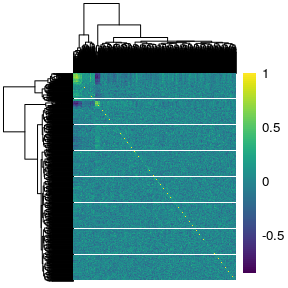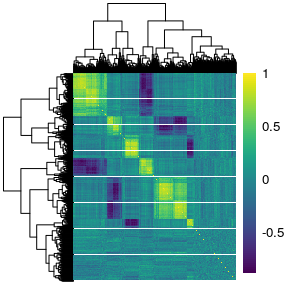## 5.2 The response The first three simulation scenarios differ in how the linear predictor $$Y^*$$ is defined, and also in the choice of regression model used to fit the data. In simulations 1 and 2 we use lasso (Tibshirani 1996) and elasticnet (Zou 2005) to fit linear models; then we use MARS (Friedman 1991) in simulation 3 to estimate non-linear effects. Simulations 4, 5 and 6 use the GLM version of these models, respectively, since the responses are binary. ### 5.2.1 Linear Relationship For simulations 1 and 2 we used the s_generate_data function to generate linear relationships beteween the response and genes, of the form: $\begin{equation} Y^* = \sum_{\substack{j\in \left\lbrace 1, \ldots, 50 \right\rbrace\\ j \in \textrm{ red, green block}}} \beta_j X_j + \beta_E E \end{equation}$ where $$\beta_j \sim \textrm{Unif}\left[ 0.9,1.1\right]$$ and . That is, only the first 50 predictors of both the red and green blocks are active. In this setting, only the main effects model is being fit to the simulated data. We used the s_generate_data with the include_interaction = TRUE argument to generate responses of the form: $\begin{equation} Y^* = \sum_{\substack{j\in \left\lbrace 1, \ldots, 50 \right\rbrace\\ j \in \textrm{ red, green block}}} \beta_j X_j + \alpha_{j} X_j E + \beta_E E \end{equation}$ where $$\beta_j \sim \textrm{Unif}\left[ 0.9,1.1\right]$$, $$\alpha_{j} \sim \textrm{Unif}\left[ 0.4,0.6\right]$$, and $$\beta_E = 2$$. In this setting, both the main effects and their interactions with E are being fit to the simulated data. In this example we generate a response which depends on both the main effects and their interactions with E. We first generate the true $$\beta$$ vector: betaMainEffect <- vector("double", length = 1000) betaMainInteractions <- vector("double", length = 1000) # the first 25 in the 3rd block are active betaMainEffect[which(truemodule1 %in% 3)[1:50]] <- runif(50, 0.9, 1.1) # the first 25 in the 4th block are active betaMainEffect[which(truemodule1 %in% 4)[1:50]] <- runif(50, 0.9, 1.1) # the interaction effects betaMainInteractions[which(betaMainEffect!=0)] <- runif(50, 0.4, 0.6) # the environment effect betaE <- 2 # the total beta vector beta <- c(betaMainEffect, betaE, betaMainInteractions) Next we run the s_generate_data function to get the necessary results for the analysis step of the simulation study. This function creates a training and a test set of equal size by evenly divinding the subjects such that there are an equal number of exposed and unexposed in both training and test sets. There are several choices to make here, but these are the most important arguments: 1. cluster_distance: How should the genes, ignoring the exposure status of the individuals, be clustered? We choose the $$TOM$$ matrix based on all subjects. 2. eclust_distance: How should the genes, accounting for the exposure status of the individuals, be clustered? We choose the difference of the exposure sensitive TOM matrices: $$TOM(X_{\textrm{diff}}) = |TOM_{E=1} - TOM_{E=0}|$$ 3. cut_method: How should the number of clusters be determined? We choose the dynamicTreeCut::cutreeDynamic() algorithm which automatically selects the number of clusters. result <- s_generate_data(p = 1000, X = X, beta = beta, include_interaction = TRUE, cluster_distance = "tom", n = 200, n0 = 100, eclust_distance = "difftom", signal_to_noise_ratio = 1, distance_method = "euclidean", cluster_method = "hclust", cut_method = "dynamic", agglomeration_method = "average", nPC = 1) ## Creating data and simulating response ## Calculating similarity matrices ## TOM calculation: adjacency.. ## adjacency: replaceMissing: 0 ## ..will not use multithreading. ## Fraction of slow calculations: 0.000000 ## ..connectivity.. ## ..matrix multiplication.. ## ..normalization.. ## ..done. ## TOM calculation: adjacency.. ## adjacency: replaceMissing: 0 ## ..will not use multithreading. ## Fraction of slow calculations: 0.000000 ## ..connectivity.. ## ..matrix multiplication.. ## ..normalization.. ## ..done. ## TOM calculation: adjacency.. ## adjacency: replaceMissing: 0 ## ..will not use multithreading. ## Fraction of slow calculations: 0.000000 ## ..connectivity.. ## ..matrix multiplication.. ## ..normalization.. ## ..done. ## Creating CV folds from training data ## Calculating number of clusters based on tom using hclust with average ## linkage and the dynamic to determine the number of clusters ## ..cutHeight not given, setting it to 0.999 ===> 99% of the (truncated) height range in dendro. ## ..done. ## Calculating number of environment clusters based on difftom ## ..cutHeight not given, setting it to 2.57 ===> 99% of the (truncated) height range in dendro. ## ..done. ## There are 2 clusters derived from the tom similarity matrix ## There are 8 clusters derived from the difftom environment similarity matrix ## There are a total of 10 clusters derived from the tom ## similarity matrix and the difftom environment similarity matrix names(result) ##  "beta_truth" "similarity" ##  "similarityEclust" "DT" ##  "Y" "X0" ##  "X1" "X_train" ##  "X_test" "Y_train" ##  "Y_test" "DT_train" ##  "DT_test" "S0" ##  "n_clusters_All" "n_clusters_Eclust" ##  "n_clusters_Addon" "clustersAll" ##  "clustersAddon" "clustersEclust" ##  "gene_groups_inter" "gene_groups_inter_Addon" ##  "tom_train_all" "tom_train_diff" ##  "tom_train_e1" "tom_train_e0" ##  "corr_train_all" "corr_train_diff" ##  "corr_train_e1" "corr_train_e0" ##  "fisherScore" "corScor" ##  "mse_null" "DT_train_folds" ##  "X_train_folds" "Y_train_folds" ### 5.2.2 Non-Linear Relationship We used the s_generate_data_mars function to generate non-linear effects of the predictors on the phenotype, of the form: $\begin{equation} Y_i^* = \sum_{\substack{j\in \left\lbrace 1, \ldots, 50 \right\rbrace\\ j \in \textrm{ red, green block}}} \beta_j X_{ij} + \beta_E E_i + \alpha_Q E_i \cdot f(Q_i) \label{eq:sim3} \end{equation}$ where \begin{align} Q_i &= - \max_{\substack{ j\in \left\lbrace 1, \ldots, 50 \right\rbrace\\ j \in \textrm{ red, green block}}} \left( X_{ij} - \bar{X}_i \right)^2 \label{eq:qiterm}\\ f(u_i) &= \frac{u_i - \displaystyle \min_{i \in \left\lbrace 1, \ldots, n \right \rbrace } u_i}{-\displaystyle \min_{i \in \left\lbrace 1, \ldots, n \right \rbrace } u_i} \label{eq:fterm}\\ \bar{X}_i &= \frac{1}{100} \sum_{\substack{j\in \left\lbrace 1, \ldots, 250 \right\rbrace\\ j \in \textrm{ red, green block}}} X_{ij} \nonumber \end{align} The s_generate_data_mars works exactly the same way as the s_generate_data function. The only difference is that the s_generate_data_mars calls the s_response_mars function to generate the response, whereas the s_generate_data function calls the s_response function to generate the response. ## 5.3 Fitting Functions In our paper, we compare three general approaches as detailed in the table below: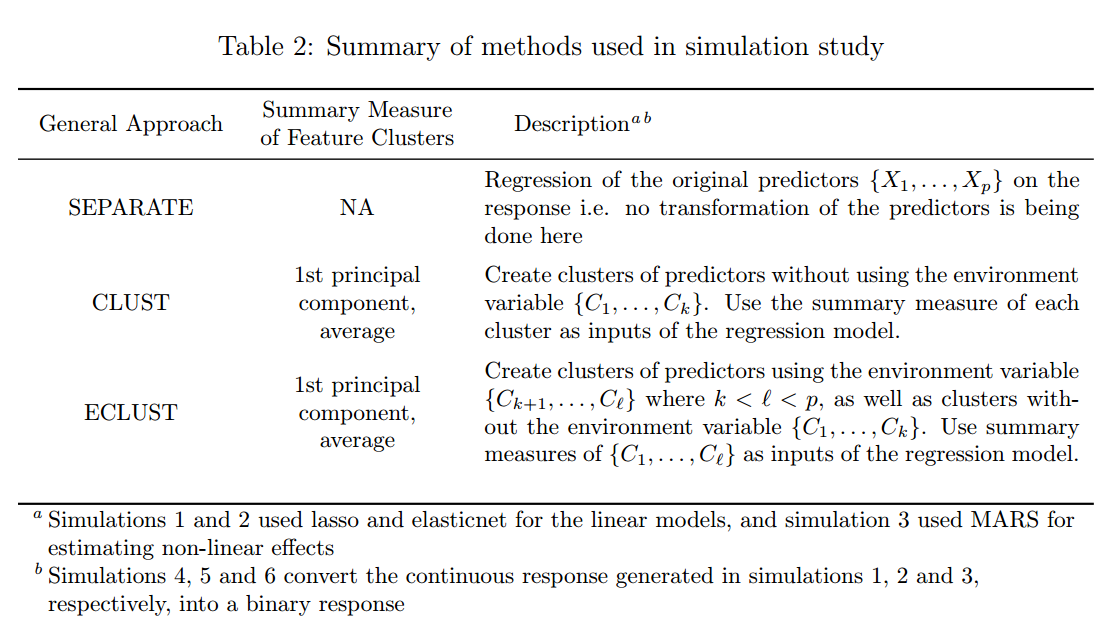There are 4 fitting functions corresponding to the approaches outlined in the table above, specifically made to be used with the simulated data: function.name General.Approach model s_pen_separate SEPARATE lasso, elasticnet, mcp, scad s_pen_clust CLUST, ECLUST lasso, elasticnet, mcp, scad s_mars_separate SEPARATE MARS s_mars_clust CLUST, ECLUST MARS In this example we fit a lasso to the clusters from the ECLUST method. The key argument here is the gene_groups. We provide result[["clustersAddon"]] to the gene_groups function because it is the clustering result using the environment information. If we wanted to run the CLUST method, then we would provide result[["clustersAll"]] to the gene_groups argument, because result[["clustersAll"]] is the clustering result from ignoring the environment information. We also specify summary = "pc" and model = "lasso" to specify that we want to use the 1st PC as the cluster representation and fit a lasso model to those clusters and their interaction with E (as specified by the include_interaction = TRUE argument) # Provide ECLUST clustering results to the gene_groups argument pen_res <- s_pen_clust(x_train = result[["X_train"]], x_test = result[["X_test"]], y_train = result[["Y_train"]], y_test = result[["Y_test"]], s0 = result[["S0"]], gene_groups = result[["clustersAddon"]], summary = "pc", model = "lasso", exp_family = "gaussian", clust_type = "ECLUST", include_interaction = TRUE) ## Summary measure: pc, Model: lasso, Cluster Type: ECLUST unlist(pen_res) ## ECLUST_pc_lasso_yes_mse ## 1800.81005 ## ECLUST_pc_lasso_yes_RMSE ## 42.43595 ## ECLUST_pc_lasso_yes_Shat ## 451.00000 ## ECLUST_pc_lasso_yes_TPR ## 0.50249 ## ECLUST_pc_lasso_yes_FPR ## 0.19444 ## ECLUST_pc_lasso_yes_CorrectSparsity ## 0.77511 ## ECLUST_pc_lasso_yes_CorrectZeroMain ## 0.69778 ## ECLUST_pc_lasso_yes_CorrectZeroInter ## 0.91333 ## ECLUST_pc_lasso_yes_IncorrectZeroMain ## 0.48515 ## ECLUST_pc_lasso_yes_IncorrectZeroInter ## 0.51000 ## ECLUST_pc_lasso_yes_nclusters ## 10.00000 The table below describes the measures of performance: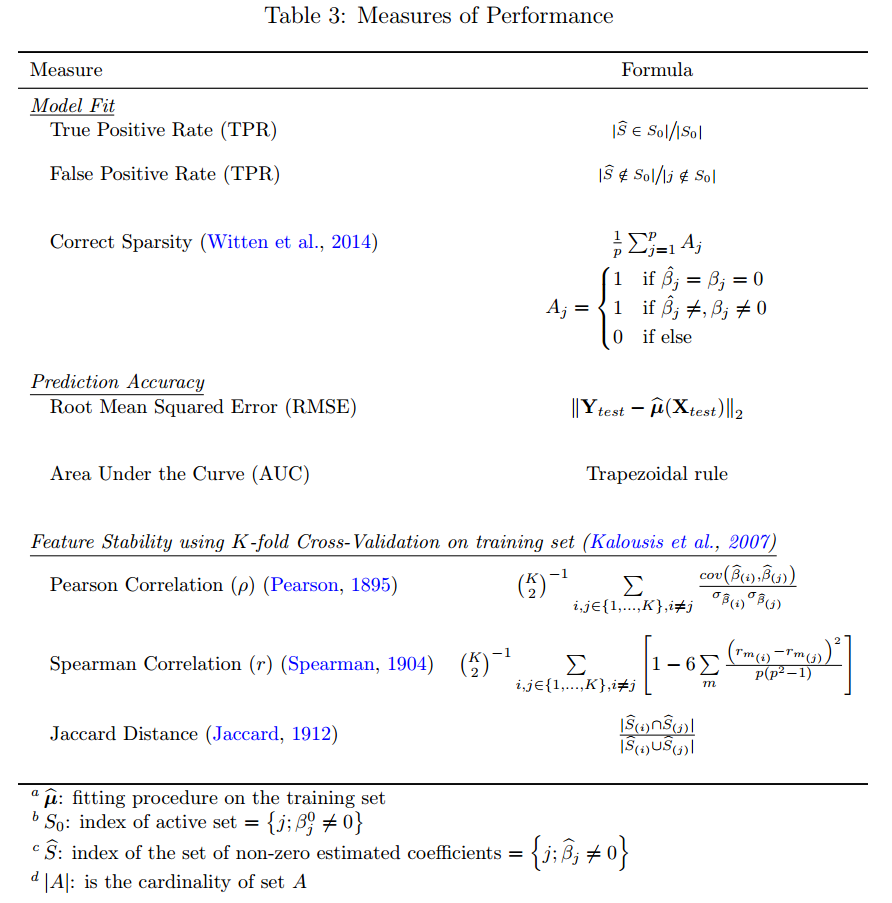# 6 Plots ## 6.1 Plot Method for Object of class similarity There is a plot method for similarity matrices included in this package, though it is very specific to the simulated data only since the resulting plot annotates the true cluster membership of the genes. The plot uses the pheatmap package for the heatmaps along with the viridis package for the color scheme so these packages need to be installed prior to using this function. The plot method is for objects of class similarity. The following objects, which are outputs of the s_generate_data function, are objects of class similarity: object.name tom_train_all tom_train_diff tom_train_e1 tom_train_e0 corr_train_all corr_train_diff corr_train_e1 corr_train_e0 fisherScore corScor To plot the heatmap of the similarity matrix, you need to provide it with the clustering tree, the cluster membership and the genes active in the response. In this example we plot the TOM matrix for the exposed subjects given by the tom_train_e1 object. The resulting heatmap has annotations for the cluster membership and if the gene is active in the response: # check that the object is of class similarity class(resulttom_train_e1)
##  "matrix"     "similarity"
# get clustering tree
hc <- hclust(as.dist(1 - result$tom_train_e1), method = "average") plot(result$tom_train_e1,
truemodule = truemodule1,
cluster_rows = hc,
cluster_cols = hc,
active = as.numeric(betaMainEffect!=0))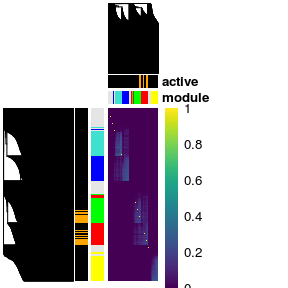## 6.2 Plot Method for Object of class eclust

There is also a function that plots heatmaps of cluster summaries such as the 1st principal component or average by exposure status. This is a plot method for object of class eclust returned by the r_cluster_data function. Two heatmaps, side-by-side are returned, where the first heatmap corresponds to the unexposed subjects and the second heatmap corresponds to the exposed subjects.

We check the class of the object returned from the clustering results on the tcgaov data:

class(cluster_res)
##  "eclust"

We simply pass this object to the generic plot function:

plot(cluster_res, show_column_names = FALSE)